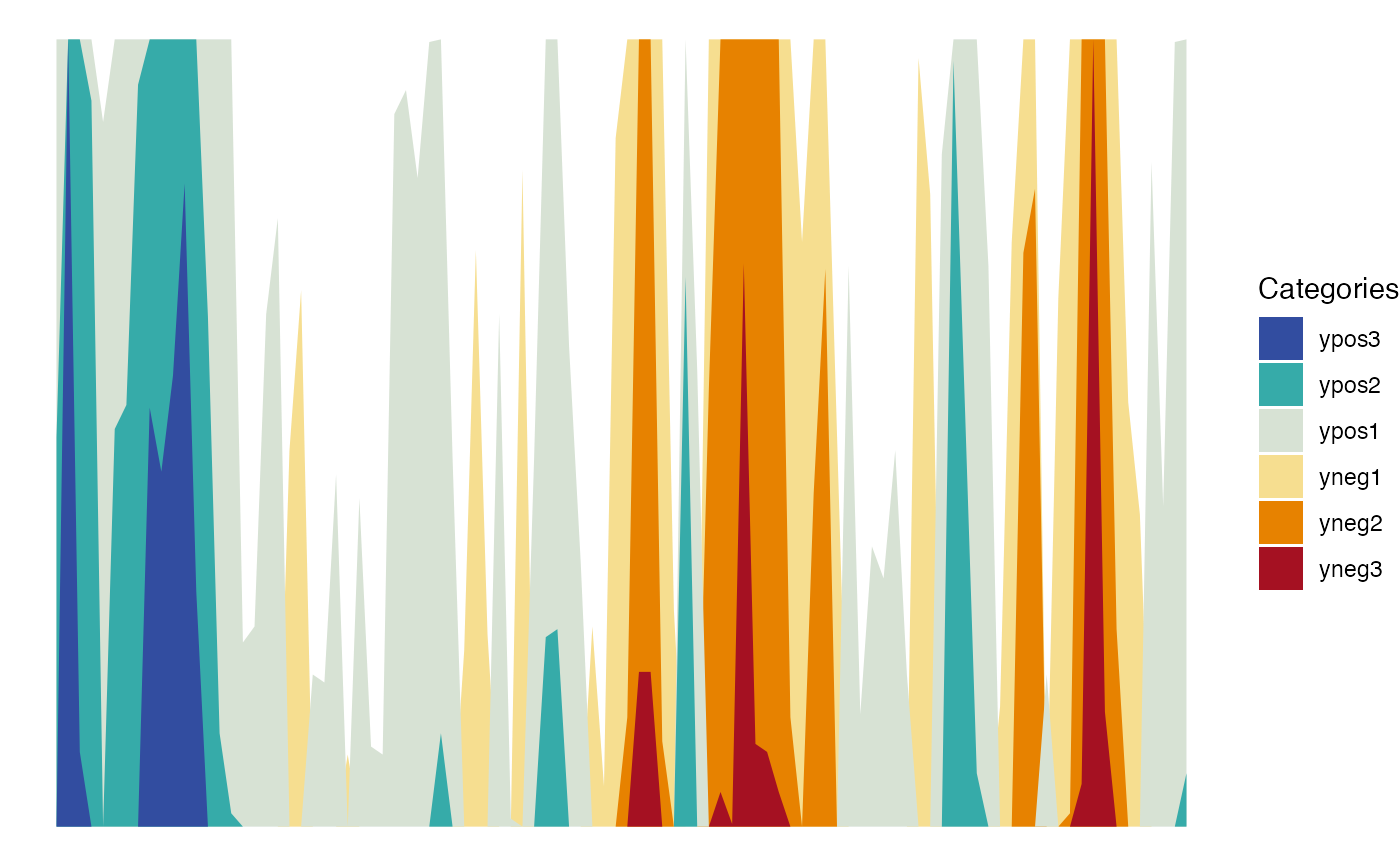This function builds horizon plots in ggplot2. It allows for the customization of the origin and the horizon scale.

geom_horizon(
mapping = NULL,
data = NULL,
position = "identity",
...,
na.rm = FALSE,
show.legend = TRUE,
origin = "midpoint",
horizonscale = 6,
rm.outliers = FALSE,
reverse = FALSE,
mirror = FALSE,
inherit.aes = TRUE
)

stat_horizon(
mapping = NULL,
data = NULL,
geom = "ribbon",
position = "identity",
...,
na.rm = FALSE,
show.legend = TRUE,
inherit.aes = TRUE,
origin = "midpoint",
horizonscale = 6,
rm.outliers = FALSE,
reverse = FALSE,
mirror = FALSE
)

## Arguments

mapping Set of aesthetic mappings created by aes() or aes_(). If specified and inherit.aes = TRUE (the default), it is combined with the default mapping at the top level of the plot. You must supply mapping if there is no plot mapping. The data to be displayed in this layer. There are three options: If NULL, the default, the data is inherited from the plot data as specified in the call to ggplot(). A data.frame, or other object, will override the plot data. All objects will be fortified to produce a data frame. See fortify() for which variables will be created. A function will be called with a single argument, the plot data. The return value must be a data.frame, and will be used as the layer data. A function can be created from a formula (e.g. ~ head(.x, 10)). Position adjustment, either as a string, or the result of a call to a position adjustment function. Other arguments passed on to layer(). These are often aesthetics, used to set an aesthetic to a fixed value, like colour = "red" or size = 3. They may also be parameters to the paired geom/stat. If FALSE, the default, missing values are removed with a warning. If TRUE, missing values are silently removed. logical. Should this layer be included in the legends? NA, the default, includes if any aesthetics are mapped. FALSE never includes, and TRUE always includes. It can also be a named logical vector to finely select the aesthetics to display. Origin of the horizon plot. It can either be a string, namely "midpoint" (the default), "median", "mean", "min" or "quantiles", or a user-specified number. Cutpoints of the horizon plot. It can either be an integer specifying the number of ranges (default is 6), or a user-supplied numeric vector with the cutpoints defining the different ranges. If TRUE, all the values below quantile(y, 0.25)-1.5*IQR(y) and above quantile(y, 0.75)+1.5*IQR(y) are excluded from the origin and cutpoint calculations (default is FALSE). @param reverse If TRUE, the horizon peaks for the values below the origin are reversed (default is FALSE). IF TRUE, the horizon peaks for all the values below the origin are reversed (default is FALSE). If TRUE, the horizon peaks for all the values are reversed (default is FALSE). If FALSE, overrides the default aesthetics, rather than combining with them. This is most useful for helper functions that define both data and aesthetics and shouldn't inherit behaviour from the default plot specification, e.g. borders(). Used geom, default to 'ribbon'

## Value

'ggplot2' layer for building a horizon plot.

## Details

A horizon plot is a special type of area plot in which the original data is transformed based on an origin and a horizon scale. The data is cut in different intervals, and the further the data is from the origin, the deeper its color usually is. All the intervals above the origin are then stacked on top of one another, keeping the intervals closest to the origin in the bottom and the furthest away ones on top. Likewise, the intervals below the origin are normally given a different color palette and they are stacked in a similar manner in the same area as the intervals above the origin. You can learn more about how horizon plots are built in vignette('ggHoriPlot') or at https://bernatgel.github.io/karyoploter_tutorial/Tutorial/PlotHorizon/PlotHorizon.html.

## Orientation

This geom treats each axis differently and, thus, can thus have two orientations. Often the orientation is easy to deduce from a combination of the given mappings and the types of positional scales in use. Thus, ggplot2 will by default try to guess which orientation the layer should have. Under rare circumstances, the orientation is ambiguous and guessing may fail. In that case the orientation can be specified directly using the orientation parameter, which can be either "x" or "y". The value gives the axis that the geom should run along, "x" being the default orientation you would expect for the geom.

## Aesthetics

geom_horizon() understands the following aesthetics (required aesthetics are in bold):

• x

• y

• xend

• alpha

• colour

• fill

## Examples

# Generate data
huron <- data.frame(year = 1875:1972, level = as.vector(LakeHuron))

# Basic plot with default colors
h <- ggplot(huron) + geom_horizon(aes(year, level))